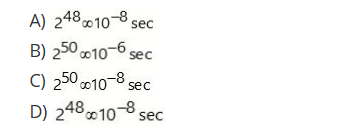141. Choose the false one. a. Diffie – Hellman protocol is a symmetric key protocol b. In RSA, both keys are private c. DES is a block clipher algorithm d. Vigenere clipher is poly-alphabetic in nature
 Answer: (b).In RSA, both keys are private

 142. There are 15 users communicating to each other. How many pairs of keys are required to authenticate the communications if the symmetric key is used? a. 15 b. 30 c. 105 d. (15)²

 143. Consider a bandwidth of line 200mbps and sequence number field is 48-bits. Find out the wrap-around time of sequence numbers.a. A b. B c. C d. D